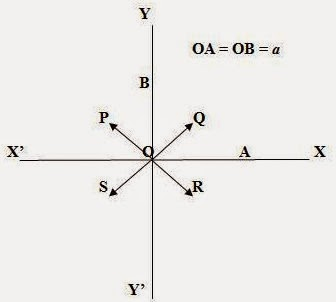## Pages

`“Life is like riding a bicycle.  To keep your balance you must keep moving.”–Albert Einstein`

## Friday, April 24, 2015

### AP Physics 2 - Multiple Choice Practice Questions Involving Magnetic Fields

“It doesn't matter how beautiful your theory is, it doesn't matter how smart you are. If it doesn't agree with experiment, it's wrong.”
– Richard Feynman

Questions involving magnetic fields are generally simple and interesting. But questions which appear simple at the first glance may tempt you to commit mistakes.

Here are a few multiple choice practice questions (single correct answer type) in this section:

(1) An electron is projected at right angles to a uniform magnetic field. The quantity that remains constant is

(a) velocity of electron

(b) momentum of electron

(c) kinetic energy of electron

(d) all the above

The velocity and momentum of the electron get changed since the direction of motion of the electron within the magnetic field changes continuously. However, the kinetic energy of the electron remains constant since the magnitudes of the velocity and momentum remain constant. Therefore, the correct option is (c).

(2) An electron is projected at right angles to a magnetic field that changes in magnitude with time. The quantity that remains constant is

(a) kinetic energy of electron

(b) momentum of electron

(c) velocity of electron

(d) none of the above

Since the magnetic field is changing, an emf is induced. The speed of the electron is changed and therefore the velocity, momentum and the kinetic energy are changed. The correct option is (d).

[Note that the particle accelerator betatron works on the principle of the production of an accelerating emf due to a changing magnetic field](3) Two very long straight conductors are arranged perpendicular to the XY plane. Their mid points are at A and B and are at the same distance ‘a’ from the origin (Fig.). Each conductor carries a current ‘I’ in the negative z-direction. What is the direction of the resultant magnetic field at the origin?

(a) along OP

(b) along OQ

(c) along OR

(d) along OS

The magnetic field produced at the origin O by the current in the conductor located at A is directed along OY.

[Note that the current is directed normally into the plane of the figure. You may use Maxwell’s cork screw rule or the right hand palm rule to obtain the direction of the magnetic field].

The magnetic field produced at the origin O by the current in the conductor located at B is directed along OX’. Since the magnetic fields produced at O by the two conductors are of the same magnitude, the resultant magnetic field at O is equally inclined to OY and OX’ and is directed along OP.

4) In question No. (3) the magnitude of the resultant magnetic flux density at the origin O is

(a) μ0 I/2πa

(b) (√2) μ0 I/2πa

(c) μ0 Ia

(d) 2 μ0 Ia

The magnetic flux density at distance a from a long conductor carrying current I is μ0 I/2πa. Therefore, the resultant magnetic flux density at the origin O due to the two flux densities directed along OY and OX’ which are at right angles is √[( μ0 I/2πa)2 + ( μ0 I/2πa)2]= (√2) μ0 I/2πa, as given in option (b).

(5) A bar magnet placed in a non-uniform magnetic field will experience

(a) a torque, but no net force

(b) a net force, but no torque

(c) neither a net force nor a torque

(d) a net force as well as a torque

If a bar magnet is located in a uniform magnetic field, the poles of the magnet will experience forces of equal magnitude, but of opposite direction. The net force acting on the magnet will therefore be zero. But the two equal and opposite forces will produce a torque which will try to rotate the bar magnet. If the magnetic field is non-uniform, the forces acting on the poles of the magnet will be of unequal magnitude so that in addition to a torque, there will be a net force on the magnet [Option (d)].